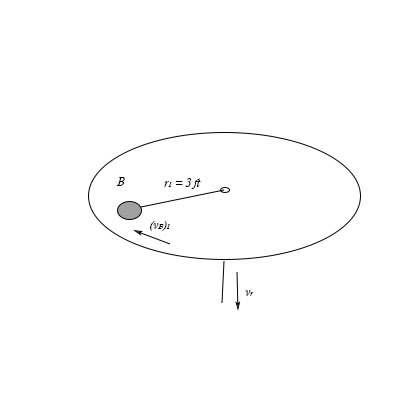# A 4 lb ball B is traveling around in a circle of radius r_1= 3 ft with a speed (v_B) = 6.0 ft/s....

## Question:

A {eq}4 lb {/eq} ball {eq}B {/eq} is traveling around in a circle of radius {eq}r_1= 3 ft {/eq} with a speed {eq}{/eq}. The attached cord is pulled down through the handle with a constant speed {eq}v_r = 2.0 ft/s {/eq}

a) Determine the bail's speed at the instant {eq}r_2 = 2 ft {/eq}. Neglect friction and the size of the ball. Express your answer to three significant figures and include the appropriate units.

b) How much work has to be done to pull down the cord at the instant {eq}r_2 = 2 ft ? {/eq} Neglect friction and the size of the ball. Express your answer to three significant figures and Include the appropriate units.## Kinetic energy:

The energy possessed by a body during its motion is known as the kinetic energy. The kinetic energy of a body depends upon the velocity with which the body is moving.

Given Data

• The weight of the ball is: {eq}W = 4\,{\rm{lb}} {/eq}
• The initial velocity of ball is: {eq}{\left( {{v_B}} \right)_1} = 6\,{\rm{ft/s}} {/eq}

(a)

The equation for conservation of angular momentum can be given as,

{eq}\begin{align*} m{\left( {{v_B}} \right)_1}{r_1}& = m{\left( {{v_B}} \right)_2}{r_2}\\ {\left( {{v_B}} \right)_1}{r_1} &= {\left( {{v_B}} \right)_2}{r_2} \end{align*} {/eq}

Here, the final velocity of ball is {eq}{\left( {{v_B}} \right)_2} {/eq}

Substitute the values in above equation,

{eq}\begin{align*} 6\,{\rm{ft/s}} \times 3\,{\rm{ft}} &= {\left( {{v_B}} \right)_2} \times 2\,{\rm{ft}}\\ {\left( {{v_B}} \right)_2}& = 9\,{\rm{ft/s}} \end{align*} {/eq}

The net final velocity can be given as,

{eq}\begin{align*} {v_{final}}& = \sqrt {\left( {{v_B}} \right)_2^2 + v_r^2} \\ {v_{final}}& = \sqrt {{{\left( 9 \right)}^2} + {{\left( 2 \right)}^2}} \\ {v_{final}}& = 9.219\,{\rm{ft/s}} \end{align*} {/eq}

(b)

The work energy equation can be written as,

{eq}\begin{align*} K.{E_1} + U &= K.{E_2}\\ \dfrac{{W\left( {{v_B}} \right)_1^2}}{{2g}} + U &= \dfrac{{Wv_{final}^2}}{{2g}} \end{align*} {/eq}

Here, the work done is {eq}U {/eq} , the initial kinetic energy is {eq}K.{E_1} {/eq} and the final kinetic energy is {eq}K.{E_2} {/eq}

Substitute the values in above equation,

{eq}\begin{align*} \dfrac{{\left( {4\,{\rm{lb}}} \right){{\left( {6\,{\rm{ft/s}}} \right)}^2}}}{{2 \times 32.2\,{\rm{ft/}}{{\rm{s}}^{\rm{2}}}}} + U &= \dfrac{{\left( {4\,{\rm{lb}}} \right){{\left( {9.219\,{\rm{ft/s}}} \right)}^2}}}{{2 \times 32.2\,{\rm{ft/}}{{\rm{s}}^{\rm{2}}}}}\\ U &= 3.04\,{\rm{lb}} \cdot {\rm{ft}} \end{align*} {/eq}Work-Energy Theorem: Definition and Application

from

Chapter 8 / Lesson 7
25K

Work and energy are closely related in physics. In this lesson, you'll learn what that relationship is as well as how we can apply it to various situations.